# Whole Number Theory

?

## Odd number

a whole number that cannot be divided exactly by 2; it has a remainder of 1.

For example, 1, 3, 5, 7, ...

1 of 17

## Even Number

a whole number that can be divided exactly by 2 (no remainder).

For example, 2, 4, 6, 8, ...

2 of 17

## Prime Number

whole number greater than 1 that can only be divided exactly between itself and 1. (A prime number only has 2 factors.)

For example, 1, 2, 3, 5, 7, ...

3 of 17

## Square Number

the product when an integer is multiplied by itself.

For example, 2 × 2 = 4, 3 × 3 = 9 and so on.

4 of 17

## Cubed Number

the product when an integer is multiplied by itself twice.

For example, 2 × 2 × 2 = 8, 3 × 3 × 3 = 27 and so on.

5 of 17

## Square Root

the number that produces a number when it is multiplied by itself.

For example, √25 is 5 because 5 × 5 = 25.

6 of 17

## Cubed Root

he number that produces a number when it is multiplied by itself and then by itself again.

For example, ∛8 is 2 because 2 × 2 × 2 = 8.

7 of 17

## Factor

number that divides exactly into another number without a remainder.

For example, factors of 6 are 1, 2, 3 and 6.

8 of 17

## Multiple

...of a number is found when you multiply that number by a whole number.

For example, multiples of 3 are 3, 6, 9, 12, ...

9 of 17

## Common Multiple

a multiple shared by 2 or more numbers.

For example, multiples of 2 are 2, 4, 6, 8, 10, 12, ... Multiples of 3 are 3, 6, 9, 12, ... 6 and 12 are common multiples of 2 and 3.

10 of 17

## Common Factor

a factor shared by 2 or more numbers. 1 is a common factor of all numbers.

For example, factors of 6 are 123 and 6. Factors of 12 are 123, 4, 6 and 12. Common factors of 6 and 12 are 1, 2, 3 and 6.

11 of 17

## Place Value Table example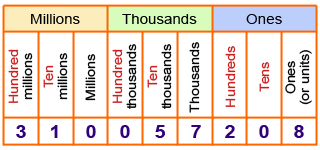12 of 17

## Prime Factors + Factor Trees

To find all the factors of a large number, you can use factor tree to list all its prime factors.

A factor tree is created by continually splitting a number into factors until only its prime factors remain.

Below are two examples of factor trees for 90.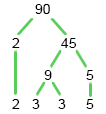OR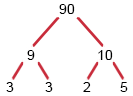Both trees show that:

90 = 2 × 3 × 3 × 5   = 2 × 32 × 5

## Use prime factors

You can find factors of large numbers using their prime factors.

##### EXAMPLE

Use the prime factors of 420 to find at least four other factors.

420 = 2 × 2 × 3 × 5 × 7

Other factors are:
2 × 2 = 4
2 × 3 = 6
2 × 5 = 10
5 × 7 = 35
2 × 3 × 5 = 30
2 × 2 × 3 × 7 = 84

13 of 17

## LCM

To find the lowest common multiple, systematically test the multiples of the largest number until you find the first one that all the other numbers go into.14 of 17

## HCF

Steps to find the HCF

1. List all the factors for each number.

2. Select the largest number that is common to all lists.

EXAMPLE 1

Find the HCF of 16 and 24.

SOLUTION

First, write down all the factors of both numbers.

NumberFactors

161, 2, 4, 8, 16

241, 2, 3, 4, 6, 8, 12, 24

The common factors are shown in red.

15 of 17

## Find lowest common multiple

The LCM of two large numbers is the larger number multiplied by any additional prime factors in the smaller number that are not common to both

##### EXAMPLE

Find the LCM of 24 and 300.

##### SOLUTION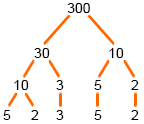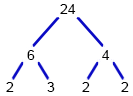Match the prime factor from the trees: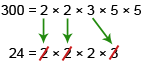The only additional prime factor for 24 is 2.

LCM = 300 × 2   = 600

16 of 17

## Find highest common factor

The HCF of two large numbers is the product of the prime factors that are common to both the numbers.

Factor trees and prime factors can be used to find the highest common factors (HCF) or lowest common multiple (LCM) of sets of numbers.

## Find highest common factor

The HCF of two large numbers is the product of the prime factors that are common to both the numbers.

##### EXAMPLE

Find the HCF of 144 and 324 by using prime factors.

##### SOLUTION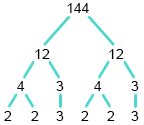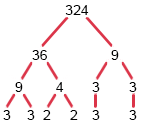From the factor trees:

144 = 2 × 2 × 2 × 2 × 3 × 3

324 = 2 × 2 × 3 × 3 × 3 × 3

The prime factors that are common to both numbers are in red. These factors are: 2, 2, 3, 3.

HCF = 2 × 2 × 3 × 3   = 36

17 of 17